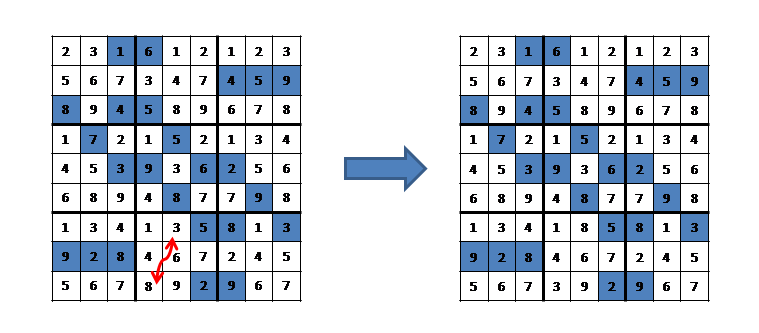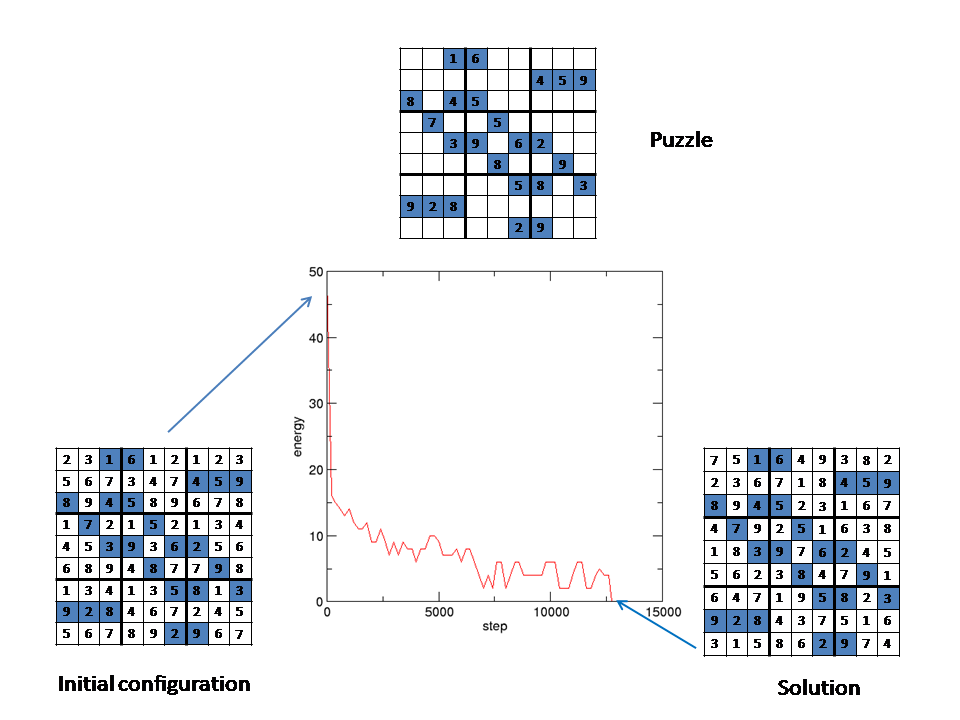# How to solve a sudoku puzzle using Monte Carlo simulationA sudoku puzzle consists of a square grid of nxn blocks each containing nxn cells. Typically, n=3. The puzzle is defined by a (variable) number of  integers in specified positions. The objective is to fill in the remaining cells so that each row, column and block contains all distinct integers from 1 to nxn once only.

Each block is examined and the open cells are filled with the remaining integers, so each square contains all nine integers.  The "energy" is defined as 243 (3n4 in general) minus the sum of the number of unique elements in each row, column and block. When the puzzle is solved, the energy is zero.

A Monte Carlo move consists of randomly selecting a pair of non-fixed elements in a randomly selected block. These elements are then exchanged to generate the trial configuration; see figure above. The trial configuation is then accepted or rejected using the standard Monte Carlo recipe. That is, if exp(-(E(trial)-E)/T) < ξ, where ξ is a uniform  random number between 0 and 1, the trial configuration is rejected.  Otherwise it is accepted.

In simulated annealing the temperature is progressively lowered until the system reaches the minimum energy configuration. In this application, however, the solution can be found at a carefully chosen constant temperature. Here is what happens with a constant temperature of 0.15. The solution, corresponding to energy=0,  is found after 12741 steps.On an Intel Core 2 Duo processor, the calcuation takes less than one second.